# SAS/ETS Examples

## A Specification Test for Non-Nested Regression Models

Contents | SAS Program

# Overview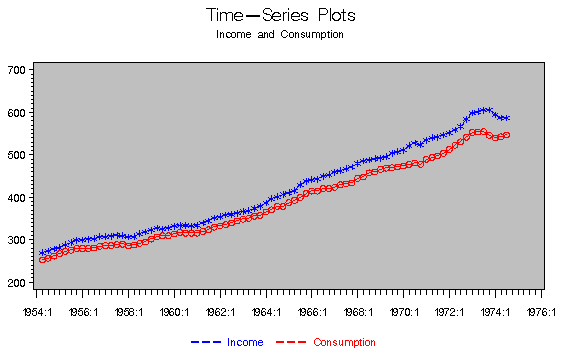In econometrics, researchers are constantly faced with the fundamental problem of choosing between models. The seminal contributions of Cox (1961) to the testing of separate families of hypotheses and Pesaran (1974) to the testing of non-nested linear regressions have lead to a burgeoning literature on the testing for non-nested models. As McAleer (1995) points out, prior to 1982, there were only about 15 published papers testing non-nested regression models. Over the last decade, however, more than 100 papers have been published.

Consider the following two models (refer to Pesaran and Deaton 1978):
where y is an (nT)x1 vector of observations on all the n dependent variables, f(·) and g(·) are the corresponding vectors of predictions, u0 and u1, of errors, and X and Z are matrices of predetermined variables. As seen above, f(·) is not nested within g(·) and vice versa. A solution consists in nesting f(·) within more general models in which the variables Z appear explicitly as exogenous or endogenous variables.

One particular way of choosing between the two hypotheses is the J-test, suggested by Davidson and Mackinnon (1981). Estimate the comprehensive model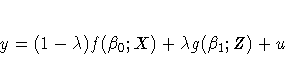note that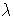is refered to as the mixing parameter hereafter). When no a priori information is available, the mixing parameter is not identifiable in the comprehensive model. The J-test works around this by replacing g(·) with the fitted values from a regression of y on Z and testing the mixing parameter for statistical significance.

This example illustrates a way to test the specification of a consumption function. The extension of this example to other non-nested models and multiple hypotheses is straightforward.

# Analysis

This example illustrates a specification problem in analyzing the relationship between consumption and income using U.S. quarterly data. One simple model postulates a linear relationship between consumption, income, and wealth; the influence of lagged income operates through the wealth term. Denoting consumption by c, income by y, and wealth by w, you postulate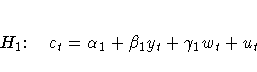where ut denotes a normally distributed disturbance vector.

An alternative is to estimate an equation containing lagged consumption as an explanatory variable. This can be thought of as a variant of Duesenberry's (1949) relative income hypothesis. The model can be postulated asAs seen above, the H1 and H2 models are non-nested.

The comprehensive models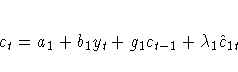and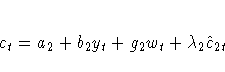whereandare the coefficients of the fitted values under H1 and H2, respectively, are both estimated. The J-test is applied by testing the mixing parameter,, for significance in each model.

The data set consists of seasonally adjusted quarterly time series of real 1958 prices, consumers' expenditure on non-durable goods and personal disposable income. These were collected from the Survey of Current Business and are used by Pesaran and Deaton (1978).

   data spec;
input yr qr c y w;
c_lag=lag(c);
date = yyq( yr, qr );
format date yyqc.;
datalines;
1954 2  253  270  0
1954 3  257  274  17
1954 4  262  279  34
1955 1  268  282  51
...
;
run;


Before the J-test can be performed, the fitted values from the two models must be calculated. The AUTOREG procedure can fit several different regressions with the inclusion of multiple model statements. In this example, two model statements are used, corresponding to the two hypotheses. For each MODEL statement an output data set is created with the OUT= option. These data sets contain the predicted values CHAT1 and CHAT2, as specified by the P= option. The DATA step merges the original data set with the two predicted data sets for use in the J-test.

    proc autoreg data=spec ;
model c = y w;              /* model for H1 */
output out=model1 p=chat1;
model c = y c_lag;          /* model for H2 */
output out=model2 p=chat2;
run;

data spec2;
set spec ;
set model1;
set model2;


Because of the limits of the J-test, it is often wise to test twice for the significance of the mixing parameter by reversing the roles of the hypotheses. The AUTOREG procedure estimates the two comprehensive models. The coefficients of CHAT1 and CHAT2 correspond to a test of the significance of the mixing parameter,. To reject a model requires that the mixing parameter be insignificantly different from 0.

    proc autoreg data=spec2;
model c = y c_lag chat1;
model c = y w chat2;
run;


The first comprehensive model yields the following parameter estimates:

 The AUTOREG Procedure

 Variable DF Estimate Standard Error t Value Approx Pr > |t| Intercept 1 8.3986 6.9758 1.20 0.2323 y 1 0.5304 0.4963 1.07 0.2885 c_lag 1 0.6349 0.0949 6.69 <.0001 chat1 1 -0.2198 0.5725 -0.38 0.7021

The estimate foris -0.2198 with a p-value of 0.7021. Thus, H1 should be rejected in favor of H2.

The second comprehensive model yields the following parameter estimates:

 The AUTOREG Procedure

 Variable DF Estimate Standard Error t Value Approx Pr > |t| Intercept 1 -3.3880 9.1509 -0.37 0.7122 y 1 -0.004955 0.1309 -0.04 0.9699 w 1 -0.001885 0.004910 -0.38 0.7021 chat2 1 1.0185 0.1522 6.69 <.0001

The estimate foris 1.0185 with a p-value of 0.0001. As it is highly significant and statistically indistinguishable from 1, it provides further support to the previous conclusion that H1 should be rejected in favor of H2.

# References

Cox, D.R. (1961), Tests of Separate Families of Hypotheses," Proceedings of the Fourth Berkeley Symposium on Mathematical Statistics and Probability, 1, Berkeley: University of California Press.

Davidson and Mackinnon (1981), Several Tests for Model Specification in the Presence of Alternative Hypotheses," Econometrica, 49, 781-793.

Duesenberry, J.S. (1949), Income, Saving, and the Theory of Consumer Behavior, Cambridge, MA: Harvard University Press.

Greene, W.H. (1993), Econometric Analysis, Second Edition, New York: Macmillan Publishing Company.

McAleer M. (1995), The Significance of Testing Empirical Non-nested Models," Journal of Econometrics, 67, 150-171.

Pesaran, M.H. (1974), On the General Problem of Model Selection," Review of Economic Studies, 41, 153-171.

Pesaran, M.H. and Deaton, A.S. (1978), Testing Non-nested Nonlinear Regression Models," Econometrica, 46, 677-694.

SAS Institute Inc. (1993), SAS/ETS Users's Guide, Version 6, Second Edition, Cary, NC: SAS Institute Inc.

Stone, J.R.E. (1973), Personal Spending and Saving in Post-War Britain," in Economic Structure and Development: Essays in Honour of Jan Tinbergen, eds. H.C. Bos, H. Linnemann, and P. De Wolff, Amsterdam: North Holland, 79-98.